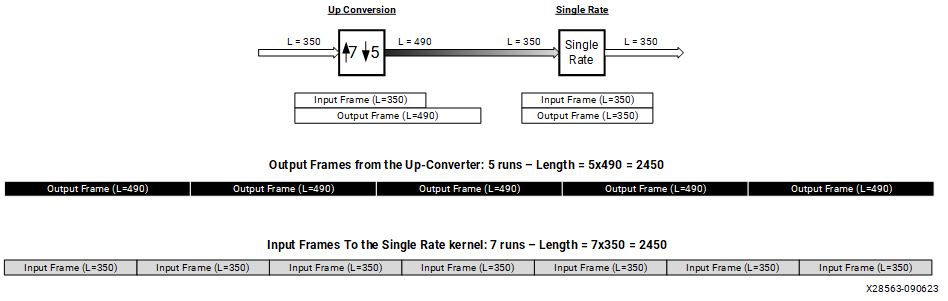# Buffer Port Connection for Multirate Processing - 2023.2 English

## AI Engine Kernel and Graph Programming Guide (UG1079)

Document ID
UG1079
Release Date
2023-10-18
Version
2023.2 English

In some designs the size of the output buffer port of a kernel can be different than the size of the input buffer port of the next kernel. In that case, the connection declaration will contain the size of the output port and the size of the input buffer port. For example:

``````connect net0 (kernel0.out, kernel1.in);
dimensions(kernel0.out) = {128};
dimensions(kernel1.in) = {192};``````

In the above example, `kernel0` writes 128 samples and `kernel1` expects 192 samples will be written to memory. In such scenarios, `aiecompiler` performs an automatic multirate analysis. In this case, `aiecompiler` will specify that `kernel1` should run twice while` kernel0` will run three times. This automatic analysis can be disabled at compile time using the flag `--disable-multirate` in the `aiecompiler` command. In that case, you must specify the repetition count for these kernels in the graph, as follows:

``````repetition_count(kernel0) = 3;
repetition_count(kernel1) = 2;``````

Just as you can multicast an output buffer port to multiple input buffer ports in the graph (automatic DMA insertion mechanism), you can also perform multirate processing in this specific use case:

``````connect net0 ( kernel0.out , kernel1.in );
connect net1 ( kernel0.out , kernel2.in );
dimensions(kernel0.out) = {128};
dimensions(kernel1.in)  = {64};
dimensions(kernel2.in)  = {192};``````

In this example, `aiecompiler` automatically detects that `kernel0` should run three times, `kernel1` should run six times, and `kernel2` should run twice for one graph iteration,` graph.run(1)`. These repetition counts can also be specified manually in the graph.

The following is a graphical example with a rational up-converter (7/5) followed by a single rate kernel. Input frame length is 350 for both, but the output frame length of the up-converter is 490.
``````connect ( datain , upconv.in );
connect ( upconv.out , singlerate.in );
connect ( singlerate.out , dataout );

// If the connection is buffer based dimensions can be specified
// in the graph
dimensions(upconv.in) = {350};
dimensions(upconv.out)  = {490};
dimensions(singlerate.in) = {350};
dimensions(singlerate.out)  = {350};

// If the connections are stream based, repetitions count must be specified
repetition_count(upconv) = 5;      // LCM(350,490)/490 = 5
repetition_count(singlerate) = 7;  // LCM(350,490)/350 = 7``````
Figure 1. Up-Converter Followed by a Single Rate KernelIn the preceding figure, the up-conversion kernel must be run five times, and the single rate kernel must be run seven times to produce and consume the same number of samples between the two kernels.

In this example the output frame length of the up-converter is larger than the length of the input frame of the single rate kernel. This means that the up-converter will write over the ping and pong buffers (or other more complex schemes) in a single iteration.